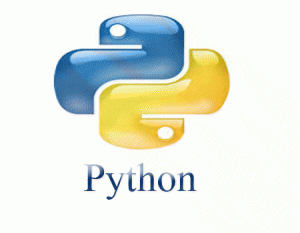## Course Fees#### Game- Making with Scratch

The module has 12 Lessons where the duration of each class is 1.5 Hours.

\$180 for 4 Lessons

Book Now#### Robotics with Mbot

The module has 12 Lessons where the duration of each class is 1.5 Hours.

\$180 for 4 Lessons

Book Now#### with Micro Bit

The module has 12 Lessons where the duration of each class is 1.5 Hours.

\$ 180 for 4 Lessons

Book Now#### Python

The module has 12 Lessons where the duration of each class is 1.5 Hours.

\$240 for 4 Lessons

Book Now#### App Development with Thunkable

The module has 12 Lessons where the duration of each class is 1.5 Hours.

\$180 for 4 Lessons

Book Now#### Scratch Jr

The module has 12 Lessons where the duration of each class is 1.5 Hours.

\$180 for 4 Lessons

Book Now

Students who opt for home-based private tuition can email us for a quote